# Community

Blog Events Webinars Tutorials Forum
×
Community Blog PostgreSQL Best Practices: Selection and Optimization of PostGIS Spatial Indexes (GiST, BRIN, and R-tree)

# PostgreSQL Best Practices: Selection and Optimization of PostGIS Spatial Indexes (GiST, BRIN, and R-tree)

This article discusses the index types supported by PostgreSQL and compares GiST and BRIN indexes' performance concerning spatial search requirements.

By Digoal

## Background

Search requirements for spatial data on PostgreSQL typically include:

1) Intersection, disjointing, or adjacency relationships between planar, 3D, or multi-dimensional objects.

2) Azimuth judgment for planar, 3D, or multi-dimensional objects (Intersects, or Strictly To The Left, Strictly To The Right, Strictly Above, or Strictly Below), which is similar to the Greater Than, Less Than, Greater Than or Equal To, and Less Than or Equal To comparisons between numerical values.

3) A planar, 3D, or multi-dimensional object contains another object.

4) A planar, 3D, or multi-dimensional object equals another object.

5) Distance between a planar, 3D, or multi-dimensional object and the boundary, nearest point, or centroid of another object. Rows meeting the criteria are returned by distance (within the specified value).

The operators are as follows: http://postgis.net/docs/manual-2.3/reference.html#Operators

``````&& — Returns TRUE if A's 2D bounding box intersects B's 2D bounding box.
&&(geometry,box2df) — Returns TRUE if a geometry's (cached) 2D bounding box intersects a 2D float precision bounding box (BOX2DF).
&&(box2df,geometry) — Returns TRUE if a 2D float precision bounding box (BOX2DF) intersects a geometry's (cached) 2D bounding box.
&&(box2df,box2df) — Returns TRUE if two 2D float precision bounding boxes (BOX2DF) intersect each other.
&&& — Returns TRUE if A's n-D bounding box intersects B's n-D bounding box.
&&&(geometry,gidx) — Returns TRUE if a geometry's (cached) n-D bounding box intersects a n-D float precision bounding box (GIDX).
&&&(gidx,geometry) — Returns TRUE if a n-D float precision bounding box (GIDX) intersects a geometry's (cached) n-D bounding box.
&&&(gidx,gidx) — Returns TRUE if two n-D float precision bounding boxes (GIDX) intersect each other.
&< — Returns TRUE if A's bounding box overlaps or is to the left of B's.
&<| — Returns TRUE if A's bounding box overlaps or is below B's.
&> — Returns TRUE if A' bounding box overlaps or is to the right of B's.
<< — Returns TRUE if A's bounding box is strictly to the left of B's.
<<| — Returns TRUE if A's bounding box is strictly below B's.
= — Returns TRUE if A's bounding box is the same as B's. Uses double precision bounding box.
>> — Returns TRUE if A's bounding box is strictly to the right of B's.
@ — Returns TRUE if A's bounding box is contained by B's.
@(geometry,box2df) — Returns TRUE if a geometry's 2D bounding box is contained into a 2D float precision bounding box (BOX2DF).
@(box2df,geometry) — Returns TRUE if a 2D float precision bounding box (BOX2DF) is contained into a geometry's 2D bounding box.
@(box2df,box2df) — Returns TRUE if a 2D float precision bounding box (BOX2DF) is contained into another 2D float precision bounding box.
|&> — Returns TRUE if A's bounding box overlaps or is above B's.
|>> — Returns TRUE if A's bounding box is strictly above B's.
~ — Returns TRUE if A's bounding box contains B's.
~(geometry,box2df) — Returns TRUE if a geometry's 2D bonding box contains a 2D float precision bounding box (GIDX).
~(box2df,geometry) — Returns TRUE if a 2D float precision bounding box (BOX2DF) contains a geometry's 2D bonding box.
~(box2df,box2df) — Returns TRUE if a 2D float precision bounding box (BOX2DF) contains another 2D float precision bounding box (BOX2DF).
~= — Returns TRUE if A's bounding box is the same as B's.
<-> — Returns the 2D distance between A and B.
|=| — Returns the distance between A and B trajectories at their closest point of approach.
<#> — Returns the 2D distance between A and B bounding boxes.
<<->> — Returns the n-D distance between the centroids of A and B bounding boxes.
<<#>> — Returns the n-D distance between A and B bounding boxes.  ``````

Do these operators support indexing? What indexing APIs are supported? How do indexes help efficiently reduce the use of operators? What is the efficiency of various indexes? What are the optimization methods for data orchestration?

## Indexes Supported by PostgreSQL

PostgreSQL supports 9 indexing APIs (additional APIs are available in practice).

### B-tree

B-Tree is undoubtedly the simplest and most easily understood index structure. KEY is classified into several hierarchies (roots, branches, and leaves) in order, and VALUE stores the actual row numbers of data.

This index structure is efficient in sorting, range search (Greater Than, Less Than, Greater Than or Equal To, and Less Than or Equal To), and exact search (Equal To) scenarios.

However, it cannot meet the search requirements based on spatial relationships of geometric objects (for example, ST_Intersects, ST_Contains, and ST_Distance) in spatial searches.

### R-tree

Spatial data is organized into nesting rectangles, with smaller ones nested in larger ones. The largest rectangle is arranged in the top layer, and the smallest in the bottom layer in descending order. This is similar to the root-branch-leaf structure of a B-tree.

Early versions of PostGIS used the PostgreSQL R-Tree indexes. Later, PostgreSQL R-trees were completely discarded, and the generalized search tree (GiST) API is used to provide faster-searching performance.

### GiST

GiST is suitable for facilitating searches for various data types, including spatial data.

During GiST indexing for GIS data, spatial data is organized in the R-Tree structure by its spatial relationships, such as "adjacency (above, below, to the left, and to the right)", "intersects", and "contains".

In addition, GIS indexes enable you to store planar, 3D, and multi-dimensional data in one index structure, which is very helpful.

### BRIN

Block range indexes (BRIN) were introduced in PostgreSQL 9.5 to store each block's boundary values (or several consecutive blocks). For example:

Numeral: Stores the maximum and minimum values of records contained in each block (or several consecutive blocks). Theoretically, you can extend BRIN indexes to contain information such as the number of records, average values, and sums. However, this leads to larger indexes.

Geometry: Stores the smallest box, which can contain the geometric objects, in records of each block (or several consecutive blocks). For example, it stores the box that comprises the lower-leftmost point and the upper-rightmost point.

## Choosing Between GiST and BRIN

### Spatial Search Requirements Supported by GiST and BRIN Indexes

#### 1) Search Requirements Supported by GiST Indexes

In addition to the preceding geometric operators, PostGIS provides many functions to support indexing.

#### 2) Search Requirements Supported by BRIN Indexes

The BRIN index stores limited information and indexes the smallest box (or multi-dimensional box) that contains all the geometric data in each block (or several consecutive blocks).

Now use BRIN to optimize the geometric searches based on the judgment of Contains, Intersects, and Disjoint.

``````&&, ~ and @ operators can be used for the 2D cases (both for "geometry" and for "geography"),

&&& operator can be used for the 3D geometries.  ``````

### Overhead of the GiST and BRIN Indexes

All indexes have an overhead. So, which of them has a larger overhead?

The answer is GiST because it stores a larger amount of information to meet more search requirements.

Example:

Code sample for creating a PostGIS extension:

``create extension postgis;  ``

Code sample for creating a test table:

``````postgres=# create table gis_idx_test(id int, info text, pos geometry) with (autovacuum_enabled=off,toast.autovacuum_enabled=off);
CREATE TABLE  ``````

#### 1) Comparison in Write Speed (10 Million Random Points)

GiST: 219s

``````postgres=# \timing
Timing is on.

create index idx_gis_idx_test on gis_idx_test using gist (pos);
insert into gis_idx_test select id, md5(random()::text), ST_SetSRID(ST_Point(180-random()*360, 90-random()*180),4326) from generate_series(1,10000000) t(id);

INSERT 0 10000000
Time: 219213.503 ms  ``````

BRIN (one box counted for each block): 33s

``````drop index idx_gis_idx_test;
truncate gis_idx_test;
create index idx_gis_idx_test on gis_idx_test using brin (pos) with (pages_per_range =1);
insert into gis_idx_test select id, md5(random()::text), ST_SetSRID(ST_Point(180-random()*360, 90-random()*180),4326) from generate_series(1,10000000) t(id);

INSERT 0 10000000
Time: 32621.684 ms  ``````

#### 2) Index-free Insertion Speed in 30s (BRIN Has Little Impact on Insertion)

``````drop index idx_gis_idx_test;
truncate gis_idx_test;
insert into gis_idx_test select id, md5(random()::text), ST_SetSRID(ST_Point(180-random()*360, 90-random()*180),4326) from generate_series(1,10000000) t(id);

INSERT 0 10000000
Time: 29696.020 ms  ``````

#### 3) Comparison in Index Creation Speed (10 Million Random Points)

GiST: 164s

``````drop index idx_gis_idx_test;
create index idx_gis_idx_test on gis_idx_test using gist (pos);

CREATE INDEX
Time: 163988.002 ms  ``````

BRIN: 3.5s

``````drop index idx_gis_idx_test;
create index idx_gis_idx_test on gis_idx_test using brin (pos) with (pages_per_range =1);

CREATE INDEX
Time: 3491.662 ms  ``````

#### 4) Comparison in Space (10 Million Random Points)

GiST: 522 MB

``````postgres=# \di+ idx_gis_idx_test
List of relations
Schema |       Name       | Type  |  Owner   |    Table     |  Size  | Description
--------+------------------+-------+----------+--------------+--------+-------------
public | idx_gis_idx_test | index | postgres | gis_idx_test | 522 MB |
(1 row)  ``````

BRIN: 5 MB

``````                                     List of relations
Schema |         Name         | Type  |  Owner   |      Table      |  Size   | Description
--------+----------------------+-------+----------+-----------------+---------+-------------
public | idx_gis_idx_test     | index | postgres | gis_idx_test    | 5136 kB |   ``````

#### 5) Comparison in Retrieval Speed

##### 5.1) Execute a Contains Query for All Points in a Polygon

GiST: 136 ms

``````postgres=# explain (analyze,verbose,timing,costs,buffers) select * from gis_idx_test where st_within (pos, ST_SetSRID(ST_MakePolygon(st_setsrid(ST_GeomFromText('LINESTRING(0 0, 15 0, 7.5 10, 0 0)'),4326)),4326));
QUERY PLAN
-------------------------------------------------------------------------------------------------------------------------------------------------------------------
Bitmap Heap Scan on public.gis_idx_test  (cost=344.25..33368.43 rows=3333 width=68) (actual time=9.180..135.562 rows=11392 loops=1)
Output: id, info, pos
Recheck Cond: ('0103000020E61000000100000004000000000000000000000000000000000000000000000000002E4000000000000000000000000000001E40000000000000244000000000000000000000000000000000'::geometry ~ gis_idx_test.pos)
Filter: _st_contains('0103000020E61000000100000004000000000000000000000000000000000000000000000000002E4000000000000000000000000000001E40000000000000244000000000000000000000000000000000'::geometry, gis_idx_test.pos)
Rows Removed by Filter: 11495
Heap Blocks: exact=20944
->  Bitmap Index Scan on idx_gis_idx_test  (cost=0.00..343.42 rows=10000 width=0) (actual time=5.550..5.550 rows=22887 loops=1)
Index Cond: ('0103000020E61000000100000004000000000000000000000000000000000000000000000000002E4000000000000000000000000000001E40000000000000244000000000000000000000000000000000'::geometry ~ gis_idx_test.pos)
Planning time: 0.187 ms
Execution time: 136.564 ms
(12 rows)  ``````

BRIN: 3.2s

9977113 rows are removed in the recheck phase, indicating that the data is in poor spatial linearity. This article discusses how to optimize this issue later.

``````postgres=# explain (analyze,verbose,timing,costs,buffers) select * from gis_idx_test where st_within (pos, ST_SetSRID(ST_MakePolygon(st_setsrid(ST_GeomFromText('LINESTRING(0 0, 15 0, 7.5 10, 0 0)'),4326)),4326));
QUERY PLAN
---------------------------------------------------------------------------------------------------------------------------------------------------
Bitmap Heap Scan on public.gis_idx_test  (cost=2631.83..35656.01 rows=3333 width=68) (actual time=67.964..3277.244 rows=11392 loops=1)
Output: id, info, pos
Recheck Cond: ('0103000020E61000000100000004000000000000000000000000000000000000000000000000002E4000000000000000000000000000001E40000000000000244000000000000000000000000000000000'::geometry ~ gis_idx_test.pos)
Rows Removed by Index Recheck: 9977113
Filter: _st_contains('0103000020E61000000100000004000000000000000000000000000000000000000000000000002E4000000000000000000000000000001E40000000000000244000000000000000000000000000000000'::geometry, gis_idx_test.pos)
Rows Removed by Filter: 11495
Heap Blocks: lossy=123457
->  Bitmap Index Scan on idx_gis_idx_test  (cost=0.00..2631.00 rows=10000 width=0) (actual time=67.473..67.473 rows=1234570 loops=1)
Index Cond: ('0103000020E61000000100000004000000000000000000000000000000000000000000000000002E4000000000000000000000000000001E40000000000000244000000000000000000000000000000000'::geometry ~ gis_idx_test.pos)
Planning time: 0.250 ms
Execution time: 3278.503 ms
(13 rows)  ``````

To improve the query efficiency of the BRIN index, you need to optimize data orchestration. For example, you need to time-serialize the scalar data (increasing the linear correlation between physical storage and logical values) and grid the spatial data because BRIN's spatial data is stored in a range box.

Next, let's proceed to optimization.

##### 5.2) Execute a KNN Query for Points Within a Radius of 100 (With the Unit Depending on the SRID) and Return the Results in the Order from Near to Far

GiST supports indexing and sorting by distance. Currently, you cannot do this with BRIN indexes. However, it supports the Contains query to construct a circle to achieve the same effect, then sort and return the filtered data.

GiST:

``````postgres=# set enable_bitmapscan=off;
SET
postgres=# explain (analyze,verbose,timing,costs,buffers) select * from gis_idx_test where ST_DWithin (pos, ST_SetSRID(ST_Point(70,10),4326), 1) order by pos <-> ST_SetSRID(ST_Point(70,10),4326);
QUERY PLAN
------------------------------------------------------------------------------------------------------------------------------------------------------------------------------
Index Scan using idx_gis_idx_test on public.gis_idx_test  (cost=0.42..1097597.75 rows=133333 width=76) (actual time=0.139..2.362 rows=476 loops=1)
Output: id, info, pos, (pos <-> '0101000020E610000000000000008051400000000000002440'::geometry)
Index Cond: (gis_idx_test.pos && '0103000020E6100000010000000500000000000000004051400000000000002240000000000040514000000000000026400000000000C0514000000000000026400000000000C05140000000000000224000000000004051400000000000002240'::geometry)
Order By: (gis_idx_test.pos <-> '0101000020E610000000000000008051400000000000002440'::geometry)
Filter: (('0101000020E610000000000000008051400000000000002440'::geometry && st_expand(gis_idx_test.pos, '1'::double precision)) AND _st_dwithin(gis_idx_test.pos, '0101000020E610000000000000008051400000000000002440'::geometry, '1'::double precision))
Rows Removed by Filter: 130
Buffers: shared hit=617
Planning time: 0.181 ms
Execution time: 2.445 ms
(9 rows)  ``````

BRIN (performance improved through data reordering according to the following section):

``````postgres=# explain (analyze,verbose,timing,costs,buffers) select * from gis_idx_test_brin where ST_DWithin (pos, ST_SetSRID(ST_Point(70,10),4326), 1) order by pos <-> ST_SetSRID(ST_Point(70,10),4326);
QUERY PLAN
-------------------------------------------------------------------------------------------------------------------------------------------------
Sort  (cost=4818.33..4818.42 rows=37 width=77) (actual time=57.087..57.144 rows=476 loops=1)
Output: id, info, pos, ((pos <-> '0101000020E610000000000000008051400000000000002440'::geometry))
Sort Key: ((gis_idx_test_brin.pos <-> '0101000020E610000000000000008051400000000000002440'::geometry))
Sort Method: quicksort  Memory: 91kB
Buffers: shared hit=836
->  Bitmap Heap Scan on public.gis_idx_test_brin  (cost=2560.18..4817.37 rows=37 width=77) (actual time=55.785..56.898 rows=476 loops=1)
Output: id, info, pos, (pos <-> '0101000020E610000000000000008051400000000000002440'::geometry)
Recheck Cond: (gis_idx_test_brin.pos && '0103000020E6100000010000000500000000000000004051400000000000002240000000000040514000000000000026400000000000C0514000000000000026400000000000C05140000000000000224000000000004051400000000000002240'::geometry)
Rows Removed by Index Recheck: 852
Filter: (('0101000020E610000000000000008051400000000000002440'::geometry && st_expand(gis_idx_test_brin.pos, '1'::double precision)) AND _st_dwithin(gis_idx_test_brin.pos, '0101000020E610000000000000008051400000000000002440'::geometry, '1'::double precision))
Rows Removed by Filter: 130
Heap Blocks: lossy=18
Buffers: shared hit=836
->  Bitmap Index Scan on idx_gis_idx_test_brin  (cost=0.00..2560.17 rows=556 width=0) (actual time=55.700..55.700 rows=180 loops=1)
Index Cond: (gis_idx_test_brin.pos && '0103000020E6100000010000000500000000000000004051400000000000002240000000000040514000000000000026400000000000C0514000000000000026400000000000C05140000000000000224000000000004051400000000000002240'::geometry)
Buffers: shared hit=818
Planning time: 0.313 ms
Execution time: 57.232 ms
(18 rows)  ``````

## Data Optimization and Planning for BRIN

To improve the query efficiency of the BRIN index, optimize the data orchestration. For example, you need to time-serialize the scalar data (increasing the linear correlation between physical storage and logical values) and grid the spatial data because BRIN's spatial data is stored in a range box.

PostGIS provides several window functions for aggregating data by the grid. Reordering data in this way improves the query efficiency of the BRIN index.

Will the BRIN index function efficiently if data is organized by grid?

Yes, there are many ways to reorder data to increase efficiency.

The following spatial data aggregation functions are applicable: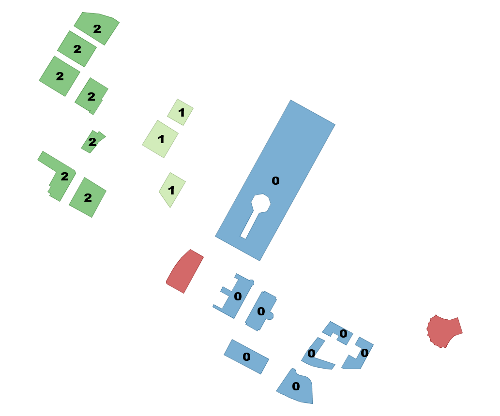Source: http://postgis.net/docs/manual-dev/ST_ClusterDBSCAN.htmlSource: http://postgis.net/docs/manual-dev/ST_ClusterKMeans.html

You can reorder the data by VALUE in Geohash:

Example:

``````postgres=# create table gis_idx_test_brin(like gis_idx_test);
CREATE TABLE
postgres=# insert into gis_idx_test_brin select * from gis_idx_test order by st_geohash(pos);
INSERT 0 10000000
postgres=# create index idx_gis_idx_test_brin on gis_idx_test_brin using brin (pos) with (pages_per_range =1);
CREATE INDEX
postgres=# explain (analyze,verbose,timing,costs,buffers) select * from gis_idx_test_brin where st_within (pos, ST_SetSRID(ST_MakePolygon(st_setsrid(ST_GeomFromText('LINESTRING(0 0, 15 0, 7.5 10, 0 0)'),4326)),4326));
QUERY PLAN
----------------------------------------------------------------------------------------------------------------------------------------------
Bitmap Heap Scan on public.gis_idx_test_brin  (cost=2631.83..35656.01 rows=3333 width=68) (actual time=56.683..76.280 rows=11392 loops=1)
Output: id, info, pos
Recheck Cond: ('0103000020E61000000100000004000000000000000000000000000000000000000000000000002E4000000000000000000000000000001E40000000000000244000000000000000000000000000000000'::geometry ~ gis_idx_test_brin.pos)
Rows Removed by Index Recheck: 2952
Filter: _st_contains('0103000020E61000000100000004000000000000000000000000000000000000000000000000002E4000000000000000000000000000001E40000000000000244000000000000000000000000000000000'::geometry, gis_idx_test_brin.pos)
Rows Removed by Filter: 11495
Heap Blocks: lossy=319
->  Bitmap Index Scan on idx_gis_idx_test_brin  (cost=0.00..2631.00 rows=10000 width=0) (actual time=56.550..56.550 rows=3190 loops=1)
Index Cond: ('0103000020E61000000100000004000000000000000000000000000000000000000000000000002E4000000000000000000000000000001E40000000000000244000000000000000000000000000000000'::geometry ~ gis_idx_test_brin.pos)
Planning time: 0.402 ms
Execution time: 77.244 ms
(13 rows)  ``````

After reordering, the response time decreases from 3278 ms to 77 ms, which is excellent.

## Examples for Indexing

### 1) GiST

The syntax for building a GiST index on a "geometry" column is as follows:

``CREATE INDEX [indexname] ON [tablename] USING GIST ( [geometryfield] ); ``

The above syntax will always build a 2D-index. To get an n-dimensional index supported in PostGIS 2.0+ for the geometry type, create one using this syntax:

``CREATE INDEX [indexname] ON [tablename] USING GIST ([geometryfield] gist_geometry_ops_nd);  ``

### 2) BRIN

The syntax for building a BRIN index on a "geometry" column is as follows:

``CREATE INDEX [indexname] ON [tablename] USING BRIN ( [geometryfield] ); ``

The above syntax always builds a 2D-index. To get a 3d-dimensional index, create one using this syntax:

``CREATE INDEX [indexname] ON [tablename] USING BRIN ([geometryfield] brin_geometry_inclusion_ops_3d);  ``

You can also get a 4d-dimensional index using the 4d operator class:

``CREATE INDEX [indexname] ON [tablename] USING BRIN ([geometryfield] brin_geometry_inclusion_ops_4d);  ``

The above syntaxes use the default number or block in a range, which is 128. To specify the number of blocks you want to summarize in a range, create one using this syntax:

``CREATE INDEX [indexname] ON [tablename] USING BRIN ( [geometryfield] ) WITH (pages_per_range = [number]);   ``

Also, keep in mind that a BRIN index only stores one index value for a large number of rows. If your table stores geometries with a mixed number of dimensions, it's likely that the resulting index will have poor performance. Avoid this drop in performance by choosing the operator class with the least number of dimensions of the stored geometries.

Also, the "geography" datatype is supported for BRIN indexing. The syntax for building a BRIN index on a "geography" column is as follows:

``CREATE INDEX [indexname] ON [tablename] USING BRIN ( [geographyfield] );   ``

## Choosing Between GiST and BRIN

To choose the index that meets your needs, consider two factors: search requirement and speed.

1) For KNN or distance-based sorting, only use GiST.

2) If geometric properties (inclusion, on the left/right/top/bottom, etc.) are involved, use either GiST or BRIN. However, to get a good filtering effect with BRIN, you need to reorder the data by the box. In this case, BRIN delivers a better result than GiST.

3) If you care about the space or data write overhead, choose BRIN (considering the spatial correlation).

## Comparing GiST and BRIN

The comparison between GiST and BRIN with 10 million spatial data records in a PostgreSQL database is described as follows:

Space: BRIN stores block-level box boundaries, therefore it requires minimal space.Write latency: BRIN has little impact on writes.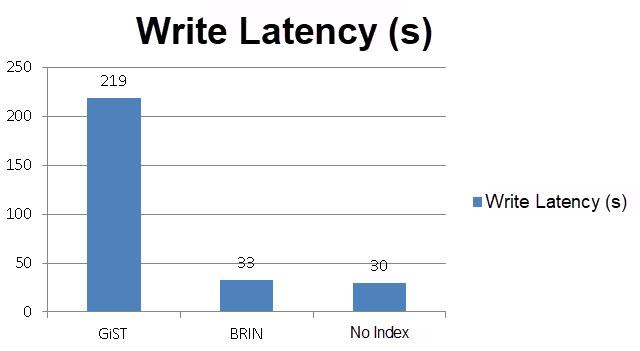Index creation latency: Creating a BRIN index is very fast.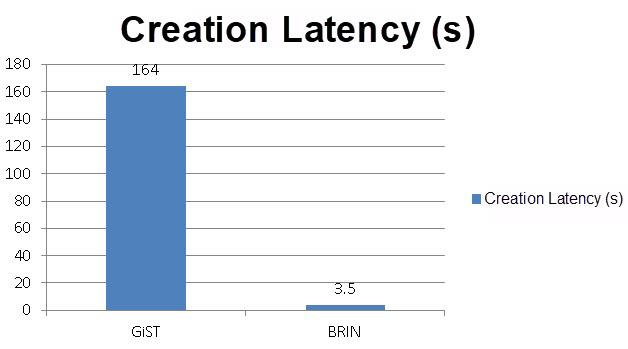Response time for executing a Contains query: BRIN provides excellent performance in facilitating KNN and Contains queries when the physical data storage space is linear.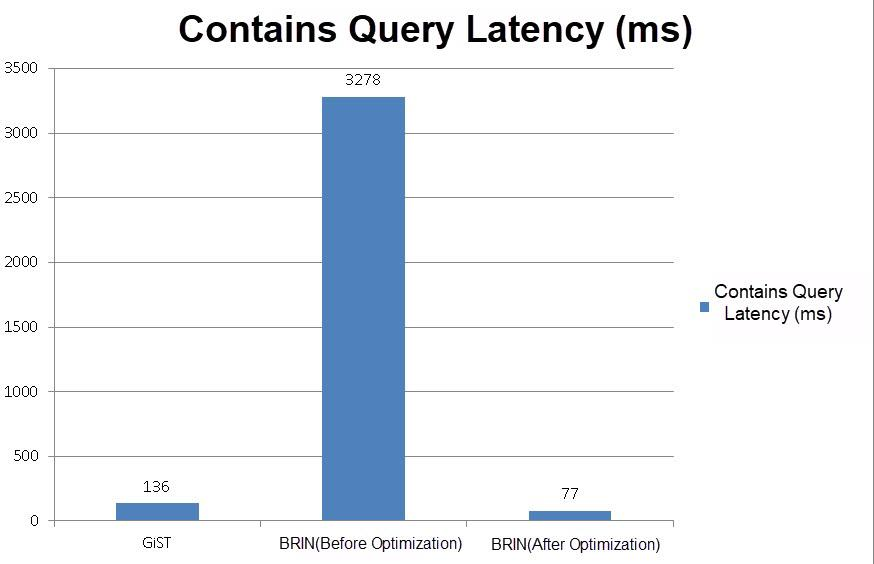KNN query performance: BRIN implements KNN queries by Contains and Sort. Its performance is slightly inferior to that of GiST.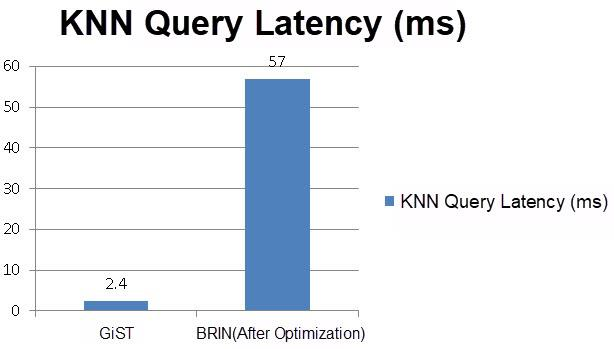## Summary

In short, BRIN is especially suitable for continuously distributed spatial data and high-speed range searches (at least one data block is returned even for point-based queries). For GIS trajectory data (historical static data), partition the data by time, reorder it by Geohash, and use the BRIN index.

For randomly distributed data, use the GIST index because it can meet more spatial data retrieval requirements. It functions well in point queries, however, it requires more space and larger write overhead. Therefore, GIST is suitable for efficient retrieval of frequently updated current status data.

0 0 0
Share on

# Related Products

• ##AnalyticDB for PostgreSQL

An online MPP warehousing service based on the Greenplum Database open source program

• ##Database Overview

Fully managed and less trouble database services

• ##AnalyticDB for MySQL

AnalyticDB for MySQL is a real-time data warehousing service that can process petabytes of data with high concurrency and low latency.

• ##ApsaraDB RDS for PostgreSQL

An on-demand database hosting service for PostgreSQL with automated monitoring, backup and disaster recovery capabilities